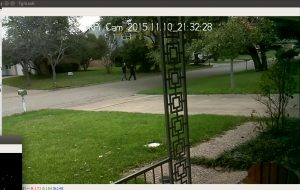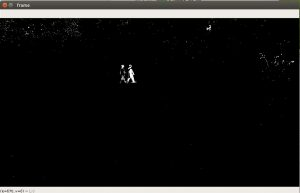# Python | Background subtraction using OpenCV

• Difficulty Level : Easy
• Last Updated : 06 Jul, 2021

Background Subtraction has several use cases in everyday life, It is being used for object segmentation, security enhancement, pedestrian tracking, counting the number of visitors, number of vehicles in traffic etc. It is able to learn and identify the foreground mask.
As the name suggests, it is able to subtract or eliminate the background portion in an image. Its output is a binary segmented image which essentially gives information about the non-stationary objects in the image. There lies a problem in this concept of finding non-stationary portion, as the shadow of the moving object can be moving and sometimes being classified in the foreground.
The popular Background subtraction algorithms are:

• BackgroundSubtractorMOG : It is a gaussian mixture based background segmentation algorithm.
• BackgroundSubtractorMOG2: It uses the same concept but the major advantage that it provides is in terms of stability even when there is change in luminosity and better identification capability of shadows in the frames.
• Geometric multigrid: It makes uses of statistical method and per pixel bayesin segmentation algorithm.

## Python3

 `# Python code for Background subtraction using OpenCV` `import` `numpy as np` `import` `cv2`   `cap ``=` `cv2.VideoCapture(``'/home/sourabh/Downloads/people-walking.mp4'``)` `fgbg ``=` `cv2.createBackgroundSubtractorMOG2()`   `while``(``1``):` `    ``ret, frame ``=` `cap.read()`   `    ``fgmask ``=` `fgbg.``apply``(frame)` ` `  `    ``cv2.imshow(``'fgmask'``, fgmask)` `    ``cv2.imshow(``'frame'``,frame )`   `    `  `    ``k ``=` `cv2.waitKey(``30``) & ``0xff` `    ``if` `k ``=``=` `27``:` `        ``break` `    `    `cap.release()` `cv2.destroyAllWindows()`

Original video frame:Background subtracted video frame:Thus, we saw an application of background subtraction algorithm detecting motions, life in video frames.

My Personal Notes arrow_drop_up
Recommended Articles
Page :